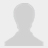/ Diigo In Education/ Group items tagged decimals

#Group items tagged

Filter: All | Bookmarks | Topics

### 6.1 Learning about Multiplication Using Dynamic Sketches of an Area Model- 29 views

• The figure below shows a rectangle with width 3 and height y. The product 3y represents the area of the 3-by-y rectangle. Change the value of y by dragging the red point up and down the vertical axis. Note that as the point is dragged, the area of the rectangle changes simultaneously.
•This is a great exploration for students to begin making connections between whole number multiplication and decimal multiplication.  One modification I would make is to have students make a partition (on grid paper) to show the partial products so I could connect the figure to a symbolic algorithm for multiplication and the distributive property.  For example, if it was 1.2 x 3, the partial products might be (1x3) + (.2 x 3).

### Mathopolis - Math Games- 175 views

1 - 20 of 38 Next ›
Showing 20 items per page